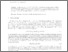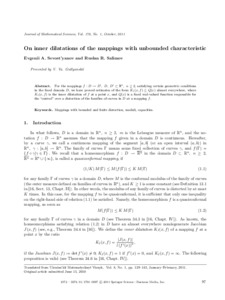# On inner dilatations of the mappings with unbounded characteristic

Sevost’yanov, Е. А. and Salіmov, R. R. (2011) On inner dilatations of the mappings with unbounded characteristic. Journal of Mathematical Sciences (178). pp. 97-107.Preview
Text

## Abstract

For the mappings f : D → D � , D, D � ⊂ Rn, n ≥ 2, satisfying certain geometric conditions in the fixed domain D, we have proved estimates of the form KI (x, f) ≤ Q(x) almost everywhere, where KI (x, f) is the inner dilatation of f at a point x, and Q(x) is a fixed real-valued function responsible for the “control” over a distortion of the families of curves in D at a mapping f.

Item Type: Article Mappings with bounded and finite distortion, moduli, capacities. Q Science > QA Mathematics > Mathematical Analysis Faculty of Physics and Mathematics > Department of Mathematical Analysis Ірина Ігорівна Таргонська 12 Nov 2014 08:20 15 Aug 2015 10:11 http://eprints.zu.edu.ua/id/eprint/13936View Item